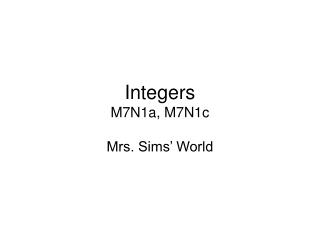DownloadDownload PresentationIntegers M7N1a, M7N1c

# Integers M7N1a, M7N1c

Télécharger la présentation## Integers M7N1a, M7N1c

- - - - - - - - - - - - - - - - - - - - - - - - - - - E N D - - - - - - - - - - - - - - - - - - - - - - - - - - -
##### Presentation Transcript

1. IntegersM7N1a, M7N1c Mrs. Sims’ World

2. What are Integers? We have learned to count by using the numbers 0, 1, 2, 3, 4, 5….. These are called whole numbers. We use whole numbers everyday in life. We use these numbers to count, to keep our checkbook, to measure temperature, and many other things. But, think about this – what do we do when the temperature falls below 0 degrees? What kind of number do we use? We use a negative number to show numbers below 0. A negative number is represented with a negative sign (also can be called a minus sign) in front of the number. Ex. -3, this number is 3 below 0 We say this as negative 3 or minus 3. All the positive numbers and negative numbers are called integers. {…, -4, -3, -2, -1, 0, 1, 2, 3, 4,…}

3. What is Absolute Value? The absolute value of a number is the distance that number is from 0 on the number line. Insert # line The absolute value of 7 is written as |7|. The answer is 7. The absolute value of -7 is written as |-7|. The answer is 7. 7 and -7 are the same distance away from 0 which is 7 spaces.

4. Adding Integers: The Rules While using negative chips and number lines may help you learn how to add integers, in reality, you need to remember the rules. Here are the rules for addition. If the signs are the same, add the numbers and keep the sign. ex. 7 + 8 = 15 (both numbers are positive) ex. -7 + -8 = -15 (both numbers are negative) If the signs are different, subtract and take the sign of the larger number. ex. -7 + 8 = 1 (I subtract. 8 is the larger number and is positive. My answer will be positive.) ex. 7 + -8 = -1 (I subtract. 8 is the larger number and is negative. My answer will be negative.)